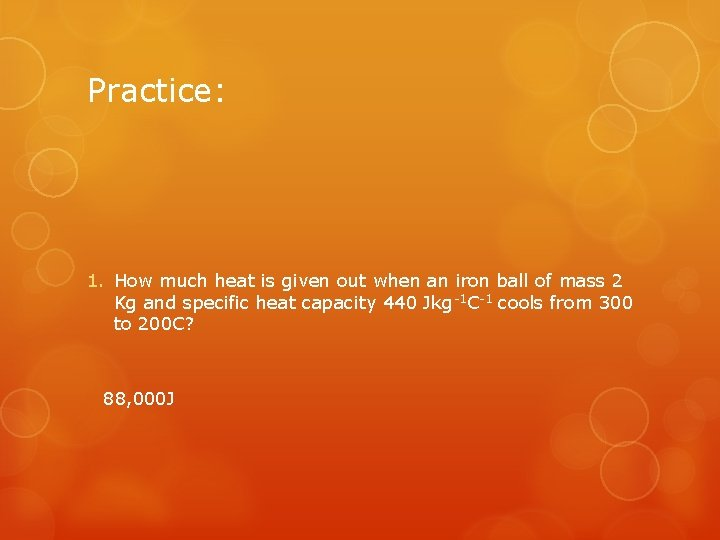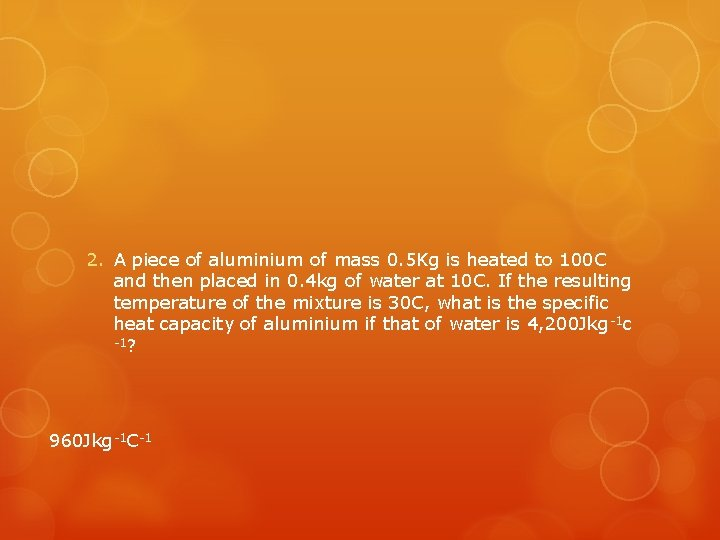# Specific heat capacity Consider 2 beakers filled with

• Slides: 8Specific heat capacityConsider 2 beaker’s filled with paraffin and water 25 c 50 C Water 75 C 25 c Hot plate paraffin If both are heated on the same heat source, over the same amount of time, the temperature rise of the paraffin will be twice as high.What is specific heat capacity? The paraffin heats up twice as much given the same amount of energy as the water does. We say that the specific heat capacity or the amount of energy it takes to raise the temperature of the water is higher than the specific heat capacity of the paraffin. Definition: “specific heat capacity of a substance is the heat used to produce unit temperature rise given unit mass” Specific heat capacity is usually quoted as the energy(j) absorbed to raise the Temperature of a mass of 1 Kg by 1 K or 1 Degree Celsius. It’s units are J Kg-1 K-1 or c-1 and it’s symbol is (c).What is specific heat capacity? E. g If a substance has a specific heat capacity of 1000 J Kg -1 C-1 Then applying 1000 J of heat energy will cause 1 kg of that substance to increase it’s temperature by 1 degree. Applying 2000 J increases the temperature by 2 C. It could also be said that applying 1000 J will cause the temperature of 0. 5 kg of that substance to rise 2 C.Please note that the specific heat capacity is also the amount of heat energy which a kilogram of that substance will release to the environment for every degree drop in temperature. E. g A substance with a specific heat capacity of 1000 Jkg 1 C-1 will release 1000 J if the temperature of 1 Kg of the substance decreases from 25 C to 24 C and 10, 000 J if it’s temperature decreases from 24 C to 14 C.The Heat equation Heat received or given out = mass x temp. change x specific heat capacity. Eheat= m x Δθ x c EH= heat energy Δ= Change of Θ= temperature c= specific heat capacityPractice: 1. How much heat is given out when an iron ball of mass 2 Kg and specific heat capacity 440 Jkg-1 C-1 cools from 300 to 200 C? 88, 000 J2. A piece of aluminium of mass 0. 5 Kg is heated to 100 C and then placed in 0. 4 kg of water at 10 C. If the resulting temperature of the mixture is 30 C, what is the specific heat capacity of aluminium if that of water is 4, 200 Jkg -1 c -1? 960 Jkg-1 C-1